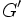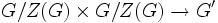# Group of nilpotency class two

## Definition

QUICK PHRASES: class two, inner automorphism group is abelian, commutator subgroup inside center, derived subgroup inside center, commutators are central, triple commutators are trivial

### Symbol-free definition

A group is said to be of nilpotency class two or nilpotence class two if it satisfies the following equivalent conditions:

1. Its nilpotency class is at most two, i.e., it is nilpotent of class at most two.
2. Its derived subgroup (i.e. commutator subgroup) is contained in its center.
3. The commutator of any two elements of the group is central.
4. Any triple commutator (i.e., a commutator where one of the terms is itself a commutator) gives the identity element.
5. Its inner automorphism group is abelian.
NOTE: nilpotency class two is occasionally used to refer to a group whose nilpotency class is precisely two, i.e., a non-abelian group whose nilpotency class is two. This is a more restrictive use of the term than the typical usage, which includes abelian groups.

VIEW: Definitions built on this | Facts about this: (facts closely related to Group of nilpotency class two, all facts related to Group of nilpotency class two) |Survey articles about this | Survey articles about definitions built on this
VIEW RELATED: Analogues of this | Variations of this | Opposites of this |
View a complete list of semi-basic definitions on this wiki
This article defines a group property: a property that can be evaluated to true/false for any given group, invariant under isomorphism
View a complete list of group properties
VIEW RELATED: Group property implications | Group property non-implications |Group metaproperty satisfactions | Group metaproperty dissatisfactions | Group property satisfactions | Group property dissatisfactions

## Examples

VIEW: groups satisfying this property | groups dissatisfying this property
VIEW: Related group property satisfactions | Related group property dissatisfactions

### Extreme examples

• The trivial group is a group of nilpotency class two (in fact, it has class zero).
• Any abelian group is a group of nilpotency class two (in fact, it has class one).

### Finite examples

By the equivalence of definitions of finite nilpotent group, every finite nilpotent group is a direct product of its Sylow subgroups. Further, if the whole group has class two, so do each of its Sylow subgroups. Thus, every finite group of nilpotency class two is obtained by taking direct products of finite groups of prime power order and class two. So, it suffices to study groups of prime power order and class two. Some salient non-abelian examples are:

• For the prime$p = 2$, dihedral group:D8 and quaternion group are two non-abelian groups of class two and order$2^3 = 8$.
• For odd primes$p$, prime-cube order group:U(3,p) and semidirect product of cyclic group of prime-square order and cyclic group of prime order are (up to isomorphism) the two non-abelian groups of order$p^3$ and class two.

## Relation with other properties

### Stronger properties

Property Meaning Proof of implication Proof of strictness (reverse implication failure) Intermediate notions
Cyclic group generated by one element Group whose automorphism group is abelian, Group whose inner automorphism group is central in automorphism group|FULL LIST, MORE INFO
Abelian group any two elements commute; center is whole group; derived subgroup is trivial; inner automorphism group is trivial CS-Baer Lie group, Frattini-in-center group, Group of nilpotency class two whose commutator map is the double of a skew-symmetric cyclicity-preserving 2-cocycle, Group of nilpotency class two whose commutator map is the double of an alternating bihomomorphism giving class two, Group of nilpotency class two whose commutator map is the skew of a cyclicity-preserving 2-cocycle, Group whose derived subgroup is contained in the square of its center, Group whose inner automorphism group is central in automorphism group, LCS-Baer Lie group|FULL LIST, MORE INFO
Group whose automorphism group is abelian automorphism group is abelian abelian automorphism group implies class two follows from class two not implies abelian automorphism group Group whose inner automorphism group is central in automorphism group|FULL LIST, MORE INFO
Group whose inner automorphism group is central in automorphism group inner automorphism group is in center of automorphism group |FULL LIST, MORE INFO
Extraspecial group Frattini-in-center group, Group of Frattini class two|FULL LIST, MORE INFO
Special group Frattini-in-center group, Group of Frattini class two|FULL LIST, MORE INFO
Frattini-in-center group commutator subgroup is contained in Frattini subgroup, which is contained in the center |FULL LIST, MORE INFO

### Weaker properties

Property Meaning Proof of implication Proof of strictness (reverse implication failure) Intermediate notions
If$G$ is nilpotent of class two, then for any$x \in G$, the map$y \mapsto [x,y]$ (or alternatively, the map$y \mapsto [y,x]$) is an endomorphism of$G$. Specifically, it is an endomorphism whose image lies inside$G'$, and we can in fact view the commutator as a biadditive map of Abelian groups:$G/Z(G) \times G/Z(G) \to G'$